## How to vlookup find the first, 2nd or nth match value in Excel?

Supposing you have two columns with Products and quantities as below screenshot shown. For quickly finding out the quantities of the first or second banana, what would you do?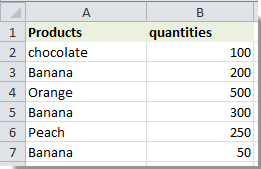Here the vlookup function can help you deal with this problem. In this article, we will show you how to vlookup find the first, second or the nth match value with the Vlookup function in Excel.

Vlookup find the first, 2nd or nth match value in Excel with formula

Easily vlookup find the first match value in Excel with Kutools for Excel

#### Vlookup find the first, 2nd or nth match value in Excel

Please do as follows to find the first, 2nd or nth match value in Excel.

1. In cell D1, enter the criteria which you want to vlookup, here I enter Banana.

2. Here we will find the first match value of banana. Select a blank cell such as E2, copy and paste formula =INDEX(\$B\$2:\$B\$6,MATCH(TRUE,EXACT(\$D\$1,\$A\$2:\$A\$6),0)) into the Formula Bar, and then press Ctrl + Shift + Enter keys simultaneously.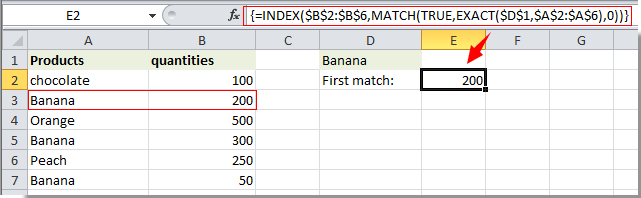Note: In this formula, \$B\$2:\$B\$6 is the range of the matching values; \$A\$2:\$A\$6 is the range with all the criteria for vlookup; \$D\$1 is the cell containing the specified vlookup criteria.

Then you will get the first match value of banana in cell E2. With this formula, you can only get the first corresponding value based on your criteria.

To get any nth relative values, you can apply the following formula: =INDEX(\$B\$2:\$B\$6,SMALL(IF(\$D\$1=\$A\$2:\$A\$6,ROW(\$A\$2:\$A\$6)-ROW(\$A\$2)+1),1)) + Ctrl + Shift + Enter keys together, this formula will return the first matched value.

Notes:

1. To find the second match value, please change the above formula to =INDEX(\$B\$2:\$B\$6,SMALL(IF(\$D\$1=\$A\$2:\$A\$6,ROW(\$A\$2:\$A\$6)-ROW(\$A\$2)+1),2)), and then press Ctrl + Shift + Enter keys simultaneously. See screenshot: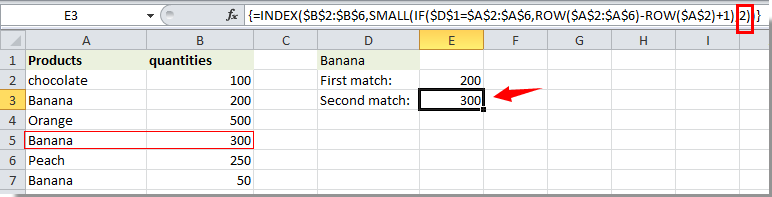2. The last number in the above formula means the nth match value of the vlookup criteria. If you change it to 3, it will get the third match value, and change to n, the nth match value will be found out.

#### Vlookup find the first match value in Excel with Kutools for Excel

You can easily find the first match value in Excel without remembering formulas with the Look for a value in list formula formula of Kutools for Excel.

1. Select a cell for locating the first matching value (says cell E2), and then click Kutools > Formula Helper > Formula Helper. See screenshot: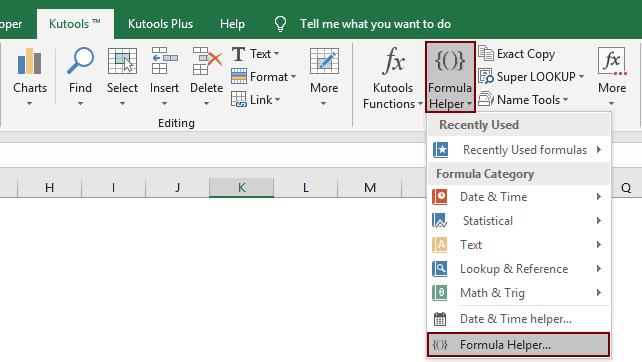3. In the Formula Helper dialog box, please configure as follows:

• 3.1 In the Choose a formula box, find and select Look for a value in list;
Tips: You can check the Filter box, enter certain word into the text box to filter the formula quickly.
• 3.2 In the Table_array box, select the table which contains the first matching value values.;
• 3.2 In the Lookup_value box, select the cell contains the criteria you will return the first value based on;
• 3.3 In the Column box, specify the column you will return the matched value from. Or you can enter the column number into the textbox directly as you need.
• 3.4 Click the OK button. See screenshot: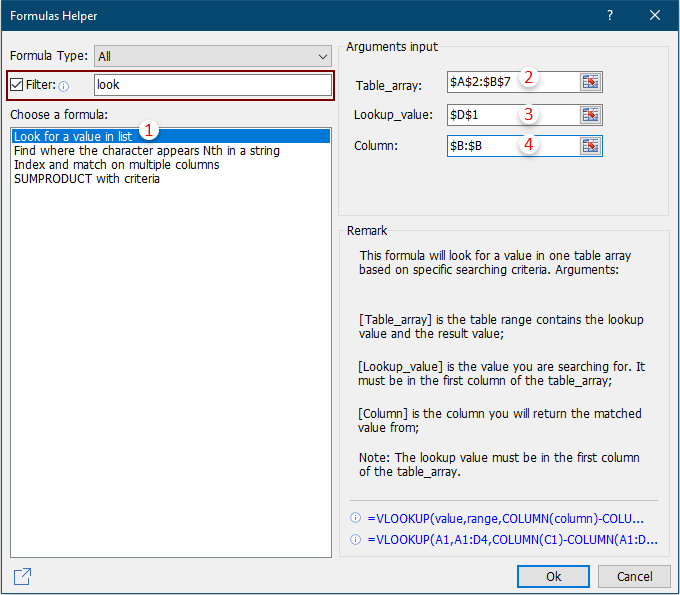Now the corresponding cell value will be auto-populated in cell C10 based on drop-down list selection.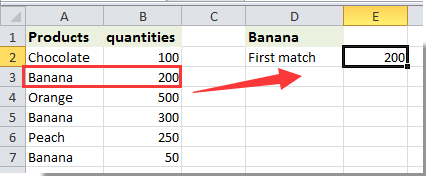If you want to have a free trial (30-day) of this utility, please click to download it, and then go to apply the operation according above steps.

### Best Office Productivity Tools

 Popular Features: Find, Highlight or Identify Duplicates   |  Delete Blank Rows   |  Combine Columns or Cells without Losing Data   |   Round without Formula ... Super Lookup: Multiple Criteria VLookup  |   Multiple Value VLookup  |   VLookup Across Multiple Sheets   |   Fuzzy Lookup .... Advanced Drop-down List: Quickly Create Drop Down List   |  Dependent Drop Down List   |  Multi-select Drop Down List .... Column Manager: Add a Specific Number of Columns  |  Move Columns  |  Toggle Visibility Status of Hidden Columns  |  Compare Ranges & Columns ... Featured Features: Grid Focus   |  Design View   |   Big Formula Bar   |  Workbook & Sheet Manager   |  Resource Library (Auto Text)   |  Date Picker   |  Combine Worksheets   |  Encrypt/Decrypt Cells   |  Send Emails by List   |  Super Filter   |   Special Filter (filter bold/italic/strikethrough...) ... Top 15 Toolsets:  12 Text Tools (Add Text, Remove Characters, ...)   |   50+ Chart Types (Gantt Chart, ...)   |   40+ Practical Formulas (Calculate age based on birthday, ...)   |   19 Insertion Tools (Insert QR Code, Insert Picture from Path, ...)   |   12 Conversion Tools (Numbers to Words, Currency Conversion, ...)   |   7 Merge & Split Tools (Advanced Combine Rows, Split Cells, ...)   |   ... and more

Supercharge Your Excel Skills with Kutools for Excel, and Experience Efficiency Like Never Before. Kutools for Excel Offers Over 300 Advanced Features to Boost Productivity and Save Time.  Click Here to Get The Feature You Need The Most...#### Office Tab Brings Tabbed interface to Office, and Make Your Work Much Easier

• Enable tabbed editing and reading in Word, Excel, PowerPoint, Publisher, Access, Visio and Project.
• Open and create multiple documents in new tabs of the same window, rather than in new windows.
• Increases your productivity by 50%, and reduces hundreds of mouse clicks for you every day!No ratings yet. Be the first to rate!
This comment was minimized by the moderator on the site
You only return first match with VLOOKUP so your article "How To Vlookup Find The First, 2nd Or Nth Match Value In Excel?" does not make any sense....
This comment was minimized by the moderator on the site
Hi Sima,
This comment was minimized by the moderator on the site
What if the next record on col b is a duplicate how do ignore duplicate? Let's Banana has quantities of 200 twice? Can you please help me figure out how to ignore the duplicate?
This comment was minimized by the moderator on the site
Cna anybody Explain the small function with the IF statement for me please? I don't really understand how that array works :(
This comment was minimized by the moderator on the site
The following formulas works perfect for me:
(a) or(b) =IF(H8='Raw Data'!B4,INDEX('Raw Data'!H6:H20,SMALL(IF(C18='Raw Data'!B6:B20,ROW('Raw Data'!B6:B20)-ROW('Raw Data'!B6:B20)+1),1)))

However, I have encountered a problem where there are 7 sets of the same criteria but in different columns:(1) can the above formula (a) be repeated and search in a different cells in a single formula and(2) the above formula (b) only allows up to two sets of the similar search with matched value result but when trying for the third set in the formula as showed below, Microsoft Excel appeared as "You've entered too many arguments for this function."
=<span style="letter-spacing: 0.2px; color: inherit; font-family: inherit; font-style: inherit; font-variant-ligatures: inherit; font-variant-caps: inherit; font-weight: inherit;">IF(H8='Raw Data'!J4,INDEX('Raw Data'!P6:P20,SMALL(IF(C18='Raw Data'!J6:J20,ROW('Raw Data'!J6:J20)-ROW('Raw Data'!J6:J20)+1),1))),</span>
This comment was minimized by the moderator on the site
What do Ctrl + Shift + Enter do?? At the beginning I didn'
This comment was minimized by the moderator on the site
Hi，An array formula needs to hit the Ctrl + Shift + Enter keys simultaneously to get the correct result.
This comment was minimized by the moderator on the site
I have a scenario... How do I get last price of anything for reference... Example: Banana first price was 200... While purchasing for second time; I need to display 200 in my expected price cell and then if I buy that on the day at 220, I will put this value manually as 220... Whenever next time I will buy banana; I need to display 220 from the last purchase price
This comment was minimized by the moderator on the site
Try =INDEX(\$B\$2:\$B\$6,XMATCH(TRUE,EXACT(\$D\$1,\$A\$2:\$A\$6),0,-1))

This is essentially reversing the search order and returning the first match using the XMATCH function.

Better late than never, hopefully helps someone :)
This comment was minimized by the moderator on the site
Perfect explanation, thanks.
This comment was minimized by the moderator on the site
If the first or any of the other entry's for 'banana' column B was blank cell, of which I don't require this number, what changes are required to this formula to skip blank cell in column B.
This comment was minimized by the moderator on the site
Sorry I am using this formula
=INDEX(\$B\$2:\$B\$6,SMALL(IF(\$D\$1=\$A\$2:\$A\$6,ROW(\$A\$2:\$A\$6)-ROW(\$A\$2)+1),1))
This comment was minimized by the moderator on the site
SOLVED:
=SMALL(IF(A2:A7=D1,IF(B2:B7<>"",B2:B7)),1)

If 2nd or 3rd number required exchange ),1) to 2 or 3

This formula does not require index, as it directly looks at the value in Cell
This comment was minimized by the moderator on the site
Correction to previous formula:
The value was reading either the lesser or greater.

Updated formula
=INDEX(\$B\$2:\$B\$7,SMALL(IF(\$A2:\$A6=\$D\$1,IF(\$B\$2:\$B\$7<>"",ROW(\$A2:\$A6)-ROW(\$A2)+1)),1))

This skips blank cell and places value of non blank cell. Replace +1 with +2 or +3 for 2nd or 3rd value
This comment was minimized by the moderator on the site
And if you want the last, second last, nth last just add a counter (count the number of events already hapenned) to the end and subtract it by 0,1,n respectively.

Thank you so much! I was searching for this for a long time
This comment was minimized by the moderator on the site
Good Day,